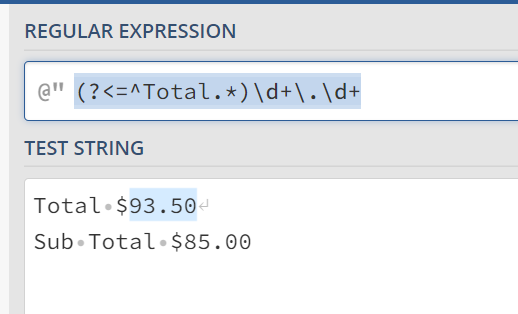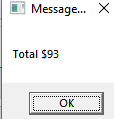# How to uniquely identify field using regex pattern?

Hi all,

I’m trying to extract the value of field “Total” using Regex Pattern of “Matches” Activity.

Fields available :
Total \$93.50
Sub Total \$85.00

Pattern I gave :
(?<=Total)(.*)
Output I’m getting:But I want to fetch only TOTAL value.

Any help would be appreciated:-)

@Vaishnav_Tej

(?<=^Total.*)\d+.\d+cheers

Hi,

Can you try the following pattern?

``````(?<=(?<!Sub\s*)Total\s*)\\$\d+
``````

OR

``````(?<=(?<!Sub\s*)Total\s*\\$)\d+
``````

Regards,

This expression is not extracting any of the values.
It just started execution and ended.

Hello sir,

This expression is not fetching decimal values.Hi,

``````(?<=(?<!Sub\s*)Total\s*)\\$[.,\d]+
``````

OR

``````(?<=(?<!Sub\s*)Total\s*\\$)[.,\d]+
``````

Regards,

Thank you sir. It’s working perfectly.

@Vaishnav_Tej

`System.Text.RegularExpressions.Regex.Matches(str,"(?<=^Total.*)\d+\.\d+",RegexOptions.Multiline)(0)`

cheers

1 Like

Actually I’m using Matches activity and inside pattern property I’m passing the expression.